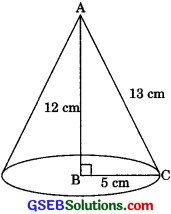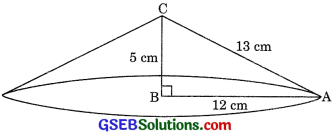# GSEB Solutions Class 9 Maths Chapter 13 Surface Areas and Volumes Ex 13.7

Gujarat Board GSEB Solutions Class 9 Maths Chapter 13 Surface Areas and Volumes Ex 13.7 Textbook Questions and Answers.

## Gujarat Board Textbook Solutions Class 9 Maths Chapter 13 Surface Areas and Volumes Ex 13.7

Question 1.
Find the volume of the right circular cone with (i) radius 6 cm, height 7 cm (ii) radius 3.5 cm, height 12 cm
Solution:
(i) r = 6 cm
h = l cm
∴ Volume of the right circular cone
= $$\frac{1}{3}$$ πr2h = $$\frac{1}{3}$$ x $$\frac{22}{7}$$ x (6)2 x 7
= 264 cm3
(ii) r = 3.5 cm
h = 12 cm
∴ Volume of the right circular cone
= $$\frac{1}{3}$$ πr2h = $$\frac{1}{3}$$ x $$\frac{22}{7}$$ x (3.5)2 x 12
= 154 cm3Question 2.
Find the capacity in litres of a conical vessel with
(i) radius 7 cm, slant height 25 cm
(ii) height 12 cm, slant height 13 cm
Solution:
(i) r = 7 cm
l = 25 cm
r2 + h2 = l2
⇒ (7)2 + h2 = (25)2
⇒ h2 = (25)2 – (7)2
⇒ h2 = 625 – 49
⇒ h2= 576
⇒ h = $$\sqrt{576}$$
⇒ h = 24 cm
∴ Capacity = $$\frac{1}{3}$$ πr2h
= $$\frac{1}{3}$$ x $$\frac{22}{7}$$ x (7)2 x 24
= 1232 cm3 = 1.232 L

(ii) h = 12 cm
l = 13 cm
r2 + h2 = l2
⇒ r2 + (12)2 = (13)2
⇒ r2 + 144 = 169
⇒ r2 = 169 – 144
⇒ r2 = 25
⇒ r = $$\sqrt{25}$$
⇒ r = 5 cm

∴ Capacity = $$\frac{1}{3}$$ πr2h
= $$\frac{1}{3}$$ x $$\frac{22}{7}$$ x (5)2 x 12
= $$\frac{2200}{7}$$ cm3 = $$\frac{2200}{7000}$$L = $$\frac{11}{35}$$ LQuestion 3.
The height of a cone is 15 cm. If its volume is 1570 cm3, find the radius of the base. (Use π = 3.14)
Solution:
Let the radius of the base of the cone be r cm.
h = 15 cm
Volume = 1570 cm3
⇒ $$\frac{1}{3}$$ πr2h x 1570
$$\frac{1}{3}$$ x 3.14 x r2 x 15 = 1570
⇒ r2 = $$\frac{1570 \times 3}{3.14 \times 15}$$
⇒ r2 = 100
⇒ r2 = 100
⇒ r = $$\sqrt{100}$$
⇒ r = 10 cm
Hence, the radius of the base of the cone is 10 cm.

Question 4.
If the volume of a right circular cone of height 9 cm is 48π cm3, find the diameter of its base.
Solution:
Let the radius of the base of the right circular
cone be r cm.
h = 9 cm
Volume = 48π cm3
⇒ $$\frac{1}{3}$$πr2h = 48π
⇒ $$\frac{1}{3}$$πr2h = 48
⇒ $$\frac{1}{3}$$ x r2 x 9 = 48
⇒ r2 = $$\frac{48 \times 3}{9}$$
⇒ r2 = 16
⇒ r2 = $$\sqrt{16}$$ = 4 cm
⇒ 2r = 2(4) = 8 cm
Hence, the diameter of the base of the right circular cone is 8 cm.Question 5.
A conical pit of top diameter 3.5 m is 12 m deep. What is its capacity in kilolitres?
Solution:
For conical pit
Diameter = 3.5 cm
∴ Radius (r) = $$\frac{3.5}{2}$$ m = 1.75 m
Depth (h) = 12 m
∴ Capacity of the conical pit
$$\frac{1}{3}$$πr2h
= $$\frac{1}{3}$$ x $$\frac{22}{7}$$ x (1.75)m2 x 12 m3
= 38.5 m3 = 38.5 x 1000L = 38.5 KL

Question 6.
The volume of a right circular cone is 9856 cm3. If the diameter of the base is 28 cm, find
(i) height of the cone,
(ii) slant height of the cone,
(iii) curved surface area of the cone.
Solution:
(i) Diameter of the base = 28 cm
∴ Radius of the base (r) = $$\frac{28}{2}$$ cm = 14 cm
Let the height of the cone be h cm.
Volume = 9856 cm3
⇒ $$\frac{1}{3}$$ πr2h = 9856
⇒ $$\frac{1}{3}$$ x $$\frac{22}{7}$$ x (14)2 x h = 9856
⇒ h = $$\frac{9856 \times 3 \times 7}{22 \times(14)^{2}}$$
⇒ h = 48 cm
Hence, the height of the cone is 48 cm.(ii) r = 14 cm
h = 48 cm
∴ I = $$\sqrt{r^{2}+h^{2}}$$ = $$\sqrt{(14)^{2}+(48)^{2}}$$
= $$\sqrt{196+2304}$$ = $$\sqrt{2500}$$ = 50 cm
Hence, the slant height of the cone is 50 cm.

(iii) r = 14 cm
l = 50 cm
∴ Curved surface area = πrl
= $$\frac{22}{7}$$ x 14 x 50 = 2200 cm2
Hence, the curved surface area of the cone is 2200 cm2.

Question 7.
A right triangle ABC with sides 5 cm, 12 cm and 13 cm is revolved about the side 12 cm. Find the volume of the solid so obtained.
Solution:
The solid obtained will be a right circular cone whose radius of the base is 5 cm and height is 12 cm.∴ r = 5 cm
h = 12 cm
∴ Volume = $$\frac{1}{3}$$ πr2h
= $$\frac{1}{3}$$ x (5)2 x 12 cm3
= 100 π cm3
Hence, the volume of the solid so obtained is 100 π cm3Question 8.
If the triangle ABC in question 7 above is revolved about the side 5 cm, then find the volume of the solid so obtained. Find also the ratio of the volumes of the two solids obtained in Questions 7 and 8.
Solution:
The solid obtained will be a right circular cone whose radius of the base is 12 cm and the height is 5 cm.
∴ r = 12 cm
h = 5 cmVolume = $$\frac{1}{3}$$πr2h
= $$\frac{1}{3}$$ x π x (12)2 x 5 cm3= 24071 cm3
The ratio of the volumes of the two solids obtained = 100π : 240 π = 5 : 12

Question 9.
A heap of wheat is in the form of a cone whose diameter is 10.5 m and height is 3 m. Find its volume. The heap is to be covered by canvas to protect it from rain. Find the area of the canvas required.
Solution:
For heap of wheat
Diameter = 10.5 m
∴ Radius (r) = $$\frac{10.5}{2}$$ cm = 5.25 m
Height (A) = 3m
∴ Volume = $$\frac{1}{3}$$πr2h
= $$\frac{1}{3}$$ x $$\frac{22}{7}$$ x (5.25)2 x 3
= 86.625 m2Slant height (l) = $$\sqrt{r^{2}+h^{2}}$$
= $$\sqrt{(5.25)^{2}+(3)^{2}}$$
= $$\sqrt{27.5625+9}$$
= $$\sqrt{36.5629}$$
= 6.05 m (approx.)
∴ Curved surface area = πrl 22
= $$\frac{22}{7}$$ x 5.25 x 6.05
= 99.825 m2
Hence, the area of the canvas required is 99.825 m2.